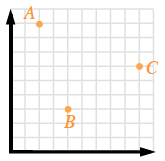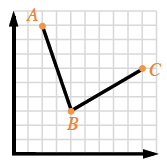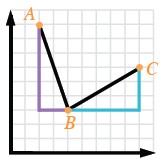### Home > CCG > Chapter Ch7 > Lesson 7.1.4 > Problem7-48

7-48.

On graph paper, graph the points $A(2,9)$, $B(4,3)$, and $C(9,6)$. Which point ($A$ or $C$) is closer to point $B$? Justify your conclusion.

Plot the points. A graph with 3 points. A (2, comma 9). B (4, comma 3). C (9, comma 6).

Draw lines to show the distance between points $A$ and $B$ and between points $C$ and $B$Draw a line from point A to B. Draw a second line from point B to C.

Draw slope triangles for both line segments. Draw a vertical line straight down from point A, 6 units, then right 2 units to connect to point B. Then drawn a horizontal line from point B, right, 5 units, and then up, 3 units to connect to point C.

Use the Pythagorean Theorem to find the lengths of the line segments.